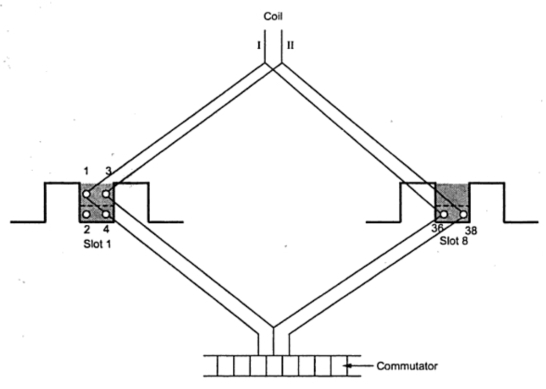### Armature Winding in D.C. Machines

The armature winding used in d.c. machines are closed and double layer. Each of the coil has its upper coil side placed at the top of one slot and lower coilside placed at the bottom of another slot which is approximately one pole pitch away.
Normally the shape of the coils is like a diamond. These coils are made in special forming machines. The back and front ends are constructed in such a way that on coilside is on a circumferentially higher level than the other. Due to this, higher coilsides are plcaed in the top of a slot while lower coilsides can be placed in the bottom of a slot.
If each slot contains two coil sides then the number of coils equal the number of slots. One coilside is there in each of top and bottom layer. But in case of low speed and high voltage machines, there are large number of coils and hence the coilsides per slot may be 4, 6, 8 or more which means 2, 3, 4 or more coilsides per layer. This is due to the fact that the number of slots can not be increased as it is difficult.
In the Fig. 1 the winding having 4 coilsides per slot are shown.Fig. 1

Note : The numbering to the coilsides is done in such a way that all the coilsides at the top are numbered odd while all the coilsides at the bottom are numbered even.
The coilside 2 is under coilside 1 while coilside 4 is under coilside 3.
1.1 Coil Span
The two coilsides of a coil are embraced by number of teeth which is known as coil sapn. The coil span is normally equal to pole pitch interms of number of teeth embraced even after deliberated chording of coils in some cases while in few cases it is not possible to have the coil span exactly equal to pole pitch. This situation comes when the number of slots are not divisible by number of poles.
Let us consider number of slots to be 25 and number of poles are say 4. A pole pitch covers 25/4 = 6.25 = 6 and 1/4 slots. Thus coil span of either 6 or 7 may be selected. Normally the nearest whole number is selected which is 6 in present case. If coil span is 6 and if top coil side of a coil lies in slot 1 then the bottom coilside will lie in 1 + 7 = 7th slot.
1.2 Pole Pitch
It may defined as the distance between the two adjacent poles i.e. the periphery of the armature divided by the number of poles. It may also be defined as the number of armature conductors or number of armature slots per pole. For example if there are if there are 64 conductors and 8 poles then pole pitch is 64/8 = 8.